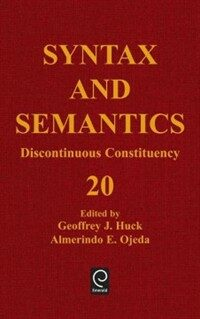> 상세정보

# 상세정보## Syntax and semantics. vol.20 : Discontinuous constituency

자료유형
단행본
개인저자
Huck, Geoffrey J, 1944-. Ojeda, Almerindo E.
단체저자명
University of Chicago. Dept. of Linguistics.
서명 / 저자사항
Syntax and semantics. vol.20 Discontinuous constituency / edited by Geoffrey J. Huck, Almerindo E. Ojeda.
발행사항
형태사항
xi, 451 p. : ill. ; 24 cm.
ISBN
0126135207 (alk. paper) 0126061017 (pbk. : alk. paper)
일반주기
Based on material presented at a conference held at the University of Chicago, July 1985, and sponsored by the University's Dept. of Linguistics.
서지주기
Includes bibliographies and index.
일반주제명
Grammar, Comparative and general -- Syntax. Generative grammar.
 000 01169camuu2200277 a 4500 001 000045159765 005 20050323103858 008 860812s1987 flua b 001 0 eng 020 ▼a 0126135207 (alk. paper) 020 ▼a 0126061017 (pbk. : alk. paper) 040 ▼a DLC ▼c DLC ▼d DLC ▼d 244002 050 0 0 ▼a P1 ▼b .S9 vol. 20 ▼a P29 082 0 0 ▼a 415 ▼2 19 090 ▼a 410 ▼b S992 ▼c 20 245 0 0 ▼a Syntax and semantics. ▼n vol.20 ▼p Discontinuous constituency / ▼c edited by Geoffrey J. Huck, Almerindo E. Ojeda. 260 ▼a Orlando : ▼b Academic Press, ▼c 1987. 300 ▼a xi, 451 p. : ▼b ill. ; ▼c 24 cm. 500 ▼a Based on material presented at a conference held at the University of Chicago, July 1985, and sponsored by the University's Dept. of Linguistics. 504 ▼a Includes bibliographies and index. 650 0 ▼a Grammar, Comparative and general ▼x Syntax. 650 0 ▼a Generative grammar. 700 1 ▼a Huck, Geoffrey J, ▼d 1944-. 700 1 ▼a Ojeda, Almerindo E. 710 2 ▼a University of Chicago. ▼b Dept. of Linguistics.

### 소장정보

No. 소장처 청구기호 등록번호 도서상태 반납예정일 예약 서비스
No. 1 소장처 청구기호 410 S992 20 등록번호 452034440 도서상태 대출가능 반납예정일 예약 서비스

### 컨텐츠정보

#### 목차


CONTENTS
Preface = xi
Introduction / GEOFFREY J. HUCK ; ALMERINDO E. OJEDA
Text = 1
Summary of Contents = 6
References = 9
Degree Complements / MARK R. BALTIN
Text = 11
References = 25
Phrase Structure, Grammatical Relations, and Discontinuous Constituents / PAULINE JACOBSON
1. Introduction = 27
2. The Verb - Particle Construction = 32
3. Wrap as a Context - Free Operation = 39
4. Relative Clause Extraposition = 62
5. Conclusion = 65
References = 68
Redoing Reduplication : A Preliminary Sketch / YOSHIHISA KITAGAWA
1. Introduction = 71
2. Affixation Approach = 71
3. Theoretical Investigation = 74
4. Copying Approach = 79
5. Empirical Investigation = 88
6. Summary and Conclusion = 104
References = 105
Analyzing Extraposition in a Tree Adjoining Grammar / ANTHONY S. KROCH ; ARAVIND K. JOSHI
1. Introduction = 107
2. An Introduction to Tree Adjoining Grammar = 109
3. TAG as a Grammatical Formalism for English = 117
4. The Basic Facts of Extraposition = 126
5. A TAG Analysis of Extraposition = 134
6. Conclusion = 146
References = 148
Some Extensions of the Autolexical Approach to Structural Mismatches / STEVEN G. LAPOINTE
1. Line Crossing = 152
2. Noun Incorporation vs. Latin -que = 160
3. Intermediate Causatives = 169
4. Summary and Further Extensions = 179
References = 182
Some Additional Evidence for Discontinuity / JAMES D. MCCAWLEY
Text = 185
References = 199
Boolean Properties in the Analysis of Gapping / RICHARD T. OEHRLE
1. Gapped Sentences with Boolean Properties = 203
2. An Informal Perspective on the Analysis = 208
3. Framework = 212
4. Basic Principles of Gapping = 219
5. The Scope of Negation = 225
6. Types = 228
7. Prosody, Types, and Scope = 234
8. The "Connective" Not = 235
9. Generalization = 236
10. Review = 237
References = 238
Discontinuous Constituents in a Free Word Order Language / WILLIAM CYGRADY
1. Introduction = 241
2. A Categoriai Grammar for Korean = 242
3. Evidence from Reflexive Pronouns = 245
4. Evidence from Quantifier Interpretation = 250
5. Conclusion = 253
References = 254
Discontinuity, Multidominance, and Unbounded Dependency in Generalized Phrase Structure Grammar : Some Preliminaries / ALMERINDO E. OJEDA
1. Introduction = 257
2. Discontinuity, Multidominance, and Unbounded Dependency = 265
3. Toward Discontinuity and Multidominance = 277
References = 281
Discontinuity in Autolexical and Autosemantic Syntax / JERROLD M. SADOCK
Text = 283
Syntax - Semantics Mismatches = 291
Conclusions = 300
References = 301
Grammatical Hierarchy and Linear Precedence / IVAN A. SAG
1. Background = 304
2. Head-Driven Phrase Structure Grammar = 308
3. A Theory of ID Rules = 318
4. Linear Precedence Rules for English = 322
5. Conclusion = 336
References = 338
Constituency and Luise$$\tilde n$$o Argument Structure / SUSAN STEELE
1. The Proposal = 343
2. Simple Constituents in Luise$$\tilde n$$o = 345
3. Argument Structure = 351
4. The Subject = 357
5. Embedding = 367
6. Final Remarks = 373
References = 374
Configurational Variation in English : A Study of Extraposition and Related Matters / SUSAN U. STUCKY
1. Introduction = 377
2. The Linguistic Domain of English Extraposition and Related Matters = 382
3. Conditions on Interpretability = 400
4. Conclusion = 402
References = 403
Linear Precedence in Discontinuous Constituents : Complex Fronting in German / HANS USZKOREIT
1. The Problem = 406
2. A GPSG Analysis of German Word Order = 410
3. An Alternative Approach = 414
4. The Proposed Framework = 419
5. Implications of the Proposal = 421
References = 424
Extraposition from NP as Anaphora / KENT WITTENBURG
1. Syntactic Approaches to Extraposition = 428
2. A Reduced Role for Syntactic Bracketing = 431
3. A DRT Analysis = 433
4. Extraposition and R-Pronouns = 437
5. Extraposition and Semantic NP Types = 439
6. Conclusion = 442
References = 444
Index = 447



### 관련분야 신착자료

박철우 (2022)

#### 체계기능언어학 : Halliday의 기능문법 입문

Halliday, M. A. K (2022)

#### Empirical evidences and theoretical assumptions in functional linguistics

Asp, Elissa D (2022)